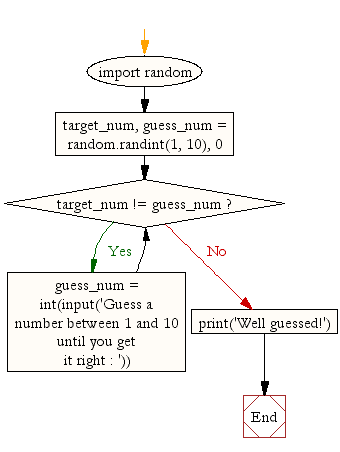﻿ Python Exercise: Guess a number between 1 to 9 - w3resource# Python Exercise: Guess a number between 1 to 9

## Python Conditional: Exercise-3 with Solution

Write a Python program to guess a number between 1 to 9.

Note : User is prompted to enter a guess. If the user guesses wrong then the prompt appears again until the guess is correct, on successful guess, user will get a "Well guessed!" message, and the program will exit.

Sample Solution:

Python Code:

``````import random
target_num, guess_num = random.randint(1, 10), 0
while target_num != guess_num:
guess_num = int(input('Guess a number between 1 and 10 until you get it right : '))
print('Well guessed!')
```
```

Sample Output:

```Guess a number between 1 and 10 until you get it right : 5
Well guessed!
```

Flowchart:## Visualize Python code execution:

The following tool visualize what the computer is doing step-by-step as it executes the said program:

Python Code Editor:

Have another way to solve this solution? Contribute your code (and comments) through Disqus.

What is the difficulty level of this exercise?

Test your Python skills with w3resource's quiz

﻿

## Python: Tips of the Day

Returns a list with n elements removed from the beginning

Example:

```def tips_take(itr, n = 1):
return itr[:n]
print(tips_take([1, 2, 3], 5))
print(tips_take([1, 2, 3], 0))
```

Output:

```[1, 2, 3]
[]
```